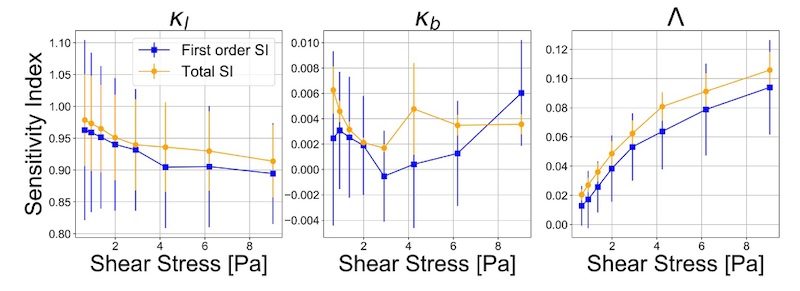In order to accurately describe the mechanics of red blood cells (RBCs) and resulting fluid dynamics, a cell-resolved blood flow fluid solver is required. The parameters of the material model for the RBC membranes are carefully tuned to reproduce the behavior of real cells under various experimental conditions. In this work, uncertainty in the parameters of the material model for RBCs used in a model for RBC suspensions was estimated with Inverse Uncertainty Quantification (IUQ) using Bayesian Annealed Sequential Importance Sampling (BASIS). Due to the relatively high computational cost of the model, a Gaussian Process regression metamodel was trained in order to feasibly draw the large number of samples required to obtain an accurate posterior distribution estimate. Additionally, the identifiability of the model parameters was estimated using Sobol sensitivity indices. The elongation index of simulated RBCs in a perfect sheared environment was the model prediction used to calibrate model parameters. The results show good identifiability of the parameter defining the tensile properties of the cell membrane and viscosity ratio, and poor identifiability of the parameter defining the response of the cell surface while undergoing bending. This suggests that the latter should be identified using a different quantity of interest. Overall, the model outputs with the optimal values of the parameters obtained using the Gaussian Process metamodel match better or close to the measurements than the results with the parameters’ values obtained with the original model. Therefore, we can conclude that it is a valid method to decrease the computational cost of IUQ of the model.

## 2020

1. Inverse uncertainty quantification of a cell model using a gaussian process metamodel
International Journal for Uncertainty Quantification, 2020